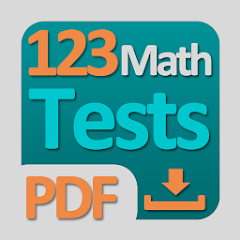# 123 Math Homework - Worksheets

1K+Everyone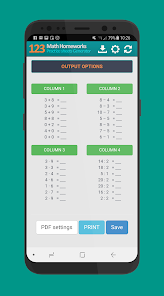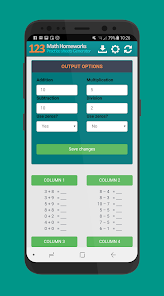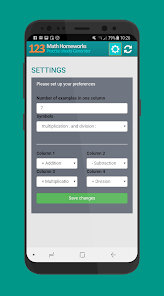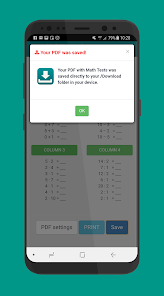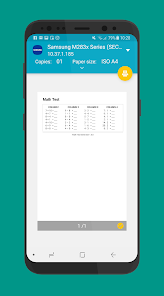123 Math Homeworks - Practice Sheets PDF Generator

Simple math tool for dynamic generation of math worksheets to test your children on multiplication, division, addition and subtraction. Simply export the prepared math homework to PDF or print directly from the app on your printer. Practice math and prepare math worksheets and homework with our app.

A great tool for parents and teachers to test the mathematics knowledge of children in the 1st to 5th grade of primary school.

Math application features

- 4 columns with math homeworks
- you can choose addition, subtraction, multiplication, division or a combination
- create your own Math test PDF
- PDF export
- unlimited number of math examples in each column
- localization of mathematical symbols
- setting the use of zeros
- setting only some numbers

This is simple tool for parents and teachers. Create and print your own math worksheets with mathematical homeworks or math tests. Train addition, subtraction, multiplication and division. You can make unlimited randomly and dynamically generated math worksheets, homeworks, tests or training sheet for printing.

Try make your own mathematical tests and homework. Print PDF! Use pencils, not only mobile application for training :) Have a fun.
Updated on
Dec 16, 2018

## Data safety

Safety starts with understanding how developers collect and share your data. Data privacy and security practices may vary based on your use, region, and age. The developer provided this information and may update it over time.No data shared with third parties